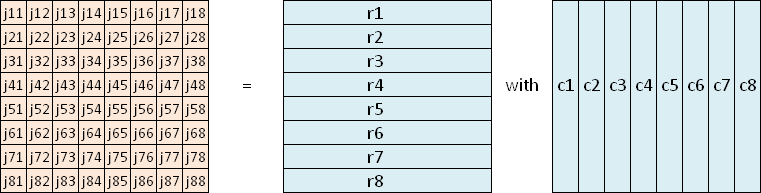Methods for calculating SM

Usually a semi-magic 8x8-square (SM) is described by 64 integers j11 to j88.
But it is also possible to describe the SM by rows r1 to r8 and columns c1 to c8.The bit vector method
Let's look at r1 as a sample for all r1 to r8 and c1 to c8:
r1 has to have the information which numbers are inside row 1.
The information about the order of the numbers is not necessary.
The numbers of a row or column are disjoint integers from the set {1,2,3,...,64}.
Therefore 64-bit-integers are able to hold the information we need.
Each bit that is set in the 64-bit-integer r1 describes a number of row 1.
For example r1 = (10000000 00000010 00000001 01000000 00000100 00010000 00100000 00001000)2 in binary format
which is equal to 264-1 + 250-1 + 241-1 + 239-1 + 227-1 + 221-1 + 214-1 + 24-1 in decimal format
means that row 1 consists of the numbers 64, 50, 41, 39, 27, 21, 14, 4
We call integers which describe a set of numbers by their bits 'bit vectors'.
(Mathematically this name is not quite correct.)
 Bit vectors and bimagic series
Only 38039 different values are possible for the bit vectors describing rows or columns,
because there are exactly 38039 bimagic series (see magic series or multimagic series).
Each SM can be transformed into another SM by any permutation of rows and by any permutation of columns.
Therefore we can demand A1 = j11 = 64. That means the number 64 is an element of row 1 and of column 1.
There are 3789 bimagic order-8 series with number 64 inside.
We call ir1 the index of r1 within the set of all 3789 bimagic series with 64 inside and ic1 the index of c1.
To avoid reflected aspects of the same unique square we additionally demand ir1 < ic1.
 How to work with the bit vectors of the rows and columns
We can use the binary operations AND, OR and XOR.
Say ri and rj describe two different rows and cn describes a column.
The result of the term ri AND rj has to be zero, because row i and row j have no common number.
If we have already determined r1, r2 and r3 we can describe the used numbers by the bit vector br3 = r1 OR r2 OR r3.
24 bits were set in the bit vector br3. The next row r4 has to fulfill the condition br3 AND r4 = 0.
You see we don't have to consider each single row that was found before.
Now look at res = ri AND cn. The result res has to be a 64-bit-integer with exactly one bit set.
To check this the SingleBit function was programmed .
 The SingleBit function
SingleBit(ri AND cn) returns 0 if ri and cn have no common bit set or more than one common bit set.
SingleBit(ri AND cn) returns the number x if ri and cn represent exactly one common number x.
If you want to calculate any SM entry, say j53 use: j53 = SingleBit(r5 AND c3)
By using the Assembly opcode bsr (searches the next bit) the SingleBit function works very fast.
An easier way to implement this function can be find in the 'tutorial program'.
 A tutorial program
The fundamental methods of the 8x8 semi-bimagic square calculation can be seen in a tutorial program.
This program calculates all 160 845 292 ess. diff. semi-magic 5x5-squares.